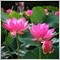# Returning a Value from the Zig Zag Indicator8

Hi,

I am calling the Zig Zag indicator with the following code but I am always getting zeros returned :-

double ZigZag= iCustom(Symbol(),0,"ZigZag", 12,5,3,1, 0);

The indicator code is below:-

I'm assuming there is something missing in the indicator to buffer or return values but I cannot work out what it is or how to do it - I have searched the forum but seem to be having the same problems with other versions.  Can anyone tell me what it is im either doing wrong or what I should be including in order to return values?

Thanks.

#property indicator_chart_window
#property indicator_buffers 1
#property indicator_color1  Red
//---- indicator parameters
input int InpDepth=12;     // Depth
input int InpDeviation=5;  // Deviation
input int InpBackstep=3;   // Backstep
//---- indicator buffers
double ExtZigzagBuffer[];
double ExtHighBuffer[];
double ExtLowBuffer[];
//--- globals
int    ExtLevel=3; // recounting's depth of extremums
//+------------------------------------------------------------------+
//| Custom indicator initialization function                         |
//+------------------------------------------------------------------+
int OnInit()
{
if(InpBackstep>=InpDepth)
{
Print("Backstep cannot be greater or equal to Depth");
return(INIT_FAILED);
}
IndicatorBuffers(3);
//---- drawing settings
SetIndexStyle(0,DRAW_SECTION);
//---- indicator buffers
SetIndexBuffer(0,ExtZigzagBuffer);
SetIndexBuffer(1,ExtHighBuffer);
SetIndexBuffer(2,ExtLowBuffer);
SetIndexEmptyValue(0,0.0);
//---- indicator short name
IndicatorShortName("ZigZag("+string(InpDepth)+","+string(InpDeviation)+","+string(InpBackstep)+")");
//---- initialization done
return(INIT_SUCCEEDED);
}
//+------------------------------------------------------------------+
//|                                                                  |
//+------------------------------------------------------------------+
int OnCalculate(const int rates_total,
const int prev_calculated,
const datetime &time[],
const double &open[],
const double &high[],
const double &low[],
const double &close[],
const long& tick_volume[],
const long& volume[],
{
int    i,limit,counterZ,whatlookfor=0;
int    back,pos,lasthighpos=0,lastlowpos=0;
double extremum;
double curlow=0.0,curhigh=0.0,lasthigh=0.0,lastlow=0.0;
//--- check for history and inputs
if(rates_total<InpDepth || InpBackstep>=InpDepth)
return(0);
//--- first calculations
if(prev_calculated==0)
limit=InitializeAll();
else
{
//--- find first extremum in the depth ExtLevel or 100 last bars
i=counterZ=0;
while(counterZ<ExtLevel && i<100)
{
if(ExtZigzagBuffer[i]!=0.0)
counterZ++;
i++;
}
//--- no extremum found - recounting all from begin
if(counterZ==0)
limit=InitializeAll();
else
{
//--- set start position to found extremum position
limit=i-1;
//--- what kind of extremum?
if(ExtLowBuffer[i]!=0.0)
{
//--- low extremum
curlow=ExtLowBuffer[i];
//--- will look for the next high extremum
whatlookfor=1;
}
else
{
//--- high extremum
curhigh=ExtHighBuffer[i];
//--- will look for the next low extremum
whatlookfor=-1;
}
//--- clear the rest data
for(i=limit-1; i>=0; i--)
{
ExtZigzagBuffer[i]=0.0;
ExtLowBuffer[i]=0.0;
ExtHighBuffer[i]=0.0;
}
}
}
//--- main loop
for(i=limit; i>=0; i--)
{
//--- find lowest low in depth of bars
extremum=low[iLowest(NULL,0,MODE_LOW,InpDepth,i)];
//--- this lowest has been found previously
if(extremum==lastlow)
extremum=0.0;
else
{
//--- new last low
lastlow=extremum;
//--- discard extremum if current low is too high
if(low[i]-extremum>InpDeviation*Point)
extremum=0.0;
else
{
//--- clear previous extremums in backstep bars
for(back=1; back<=InpBackstep; back++)
{
pos=i+back;
if(ExtLowBuffer[pos]!=0 && ExtLowBuffer[pos]>extremum)
ExtLowBuffer[pos]=0.0;
}
}
}
//--- found extremum is current low
if(low[i]==extremum)
ExtLowBuffer[i]=extremum;
else
ExtLowBuffer[i]=0.0;
//--- find highest high in depth of bars
extremum=high[iHighest(NULL,0,MODE_HIGH,InpDepth,i)];
//--- this highest has been found previously
if(extremum==lasthigh)
extremum=0.0;
else
{
//--- new last high
lasthigh=extremum;
//--- discard extremum if current high is too low
if(extremum-high[i]>InpDeviation*Point)
extremum=0.0;
else
{
//--- clear previous extremums in backstep bars
for(back=1; back<=InpBackstep; back++)
{
pos=i+back;
if(ExtHighBuffer[pos]!=0 && ExtHighBuffer[pos]<extremum)
ExtHighBuffer[pos]=0.0;
}
}
}
//--- found extremum is current high
if(high[i]==extremum)
ExtHighBuffer[i]=extremum;
else
ExtHighBuffer[i]=0.0;
}
//--- final cutting
if(whatlookfor==0)
{
lastlow=0.0;
lasthigh=0.0;
}
else
{
lastlow=curlow;
lasthigh=curhigh;
}
for(i=limit; i>=0; i--)
{
switch(whatlookfor)
{
case 0: // look for peak or lawn
if(lastlow==0.0 && lasthigh==0.0)
{
if(ExtHighBuffer[i]!=0.0)
{
lasthigh=High[i];
lasthighpos=i;
whatlookfor=-1;
ExtZigzagBuffer[i]=lasthigh;
}
if(ExtLowBuffer[i]!=0.0)
{
lastlow=Low[i];
lastlowpos=i;
whatlookfor=1;
ExtZigzagBuffer[i]=lastlow;
}
}
break;
case 1: // look for peak
if(ExtLowBuffer[i]!=0.0 && ExtLowBuffer[i]<lastlow && ExtHighBuffer[i]==0.0)
{
ExtZigzagBuffer[lastlowpos]=0.0;
lastlowpos=i;
lastlow=ExtLowBuffer[i];
ExtZigzagBuffer[i]=lastlow;
}
if(ExtHighBuffer[i]!=0.0 && ExtLowBuffer[i]==0.0)
{
lasthigh=ExtHighBuffer[i];
lasthighpos=i;
ExtZigzagBuffer[i]=lasthigh;
whatlookfor=-1;
}
break;
case -1: // look for lawn
if(ExtHighBuffer[i]!=0.0 && ExtHighBuffer[i]>lasthigh && ExtLowBuffer[i]==0.0)
{
ExtZigzagBuffer[lasthighpos]=0.0;
lasthighpos=i;
lasthigh=ExtHighBuffer[i];
ExtZigzagBuffer[i]=lasthigh;
}
if(ExtLowBuffer[i]!=0.0 && ExtHighBuffer[i]==0.0)
{
lastlow=ExtLowBuffer[i];
lastlowpos=i;
ExtZigzagBuffer[i]=lastlow;
whatlookfor=1;
}
break;
}
}

//--- done
return(rates_total);
}
//+------------------------------------------------------------------+
//|                                                                  |
//+------------------------------------------------------------------+
int InitializeAll()
{
ArrayInitialize(ExtZigzagBuffer,0.0);
ArrayInitialize(ExtHighBuffer,0.0);
ArrayInitialize(ExtLowBuffer,0.0);
//--- first counting position
return(Bars-InpDepth);
}
//+------------------------------------------------------------------+967

matt_a20:

Hi,

I am calling the Zig Zag indicator with the following code but I am always getting zeros returned :-

double ZigZag= iCustom(Symbol(),0,"ZigZag", 12,5,3,1, 0);

The indicator code is below:-

I'm assuming there is something missing in the indicator to buffer or return values but I cannot work out what it is or how to do it - I have searched the forum but seem to be having the same problems with other versions.  Can anyone tell me what it is im either doing wrong or what I should be including in order to return values?

Thanks.

You first have to find where the buffers does not equal to 0 which start from bar 0 backwards.45996

Use this function to get it.

```double ZZPeak(string a_symbol, int shift) {
bool signal=false;
double gd_result=0;
double gd_peak[]; ArrayResize(gd_peak,shift+1); ArrayInitialize(gd_peak,0);
int found=0; int c=0;
while(found<shift+1){